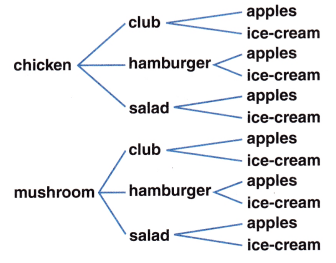# McGraw Hill Math Grade 6 Lesson 25.4 Answer Key Tree Diagrams

Practice questions available in McGraw Hill Math Grade 6 Answer Key PDF Lesson 25.4 Tree Diagrams will engage students and is a great way of informal assessment.

Exercises

INTERPRET

Question 1.
How many different outcomes are there in the tree diagram?Red – RR, RW, RG
White – WR, WW, WG
Green – GR, GW, GG
So, the different outcomes are 9 in the tree diagram.

Question 2.
Below is a tree diagram of the choices at the picnic you are going to attend. If you do not like hamburgers, how many different meal combinations can you select from?Chicken – Club – apples, ice cream
Chicken – Salad – apples, ice cream
Mushroom – Club – apples, ice cream
Mushroom – Salad – apples, ice cream
There are 4 possible outcomes.

SOLVE

Question 3.
Draw a tree diagram that shows the possible outcomes of flipping a coin 3 times.So, the possible outcomes of flipping a coin 3 times are 8.

Question 4.
How many outcomes are there where both John and Bill fail?Favorable outcomes = {(Bill passes, Bill fails), (Bill passes, Bill fails)}
So, the outcomes are 2.

Question 5.
Below is a tree diagram of the possibilities where four girls—Heather, Alice, Lucy, and Annie—each choose one item for their desk. How many different outcomes